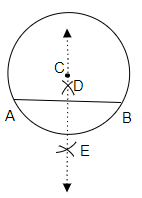# Draw a circle with centre $\mathrm{C}$ and radius $3.4 \mathrm{~cm}$. Draw any chord $\overline{\mathrm{AB}}$. Construct the perpendicular bisector of $\overline{\mathrm{AB}}$ and examine if it passes through $\mathrm{C}$.

To do:

We have to construct the perpendicular bisector of $\overline{AB}$ and examine if it passes through $C$.

Solution:Steps of construction:

(i) Let us draw a circle with centre $C$ and radius $3.4\ cm$.

(ii) Now, let us draw any chord on the circle with centre $C$ and point the intersection of the chord with the circle as $A$ and $B$ respectively.

(iii) Now, let us draw two arcs by taking $A$ and $B$ as centres above $\overline{AB}$ and let us point the point of intersection of two arcs as $D$.

(iv) Similarly let us draw two arcs by taking $A$ and $B$ as centres below $\overline{AB}$  and let us point the point of intersection of two arcs as $E$.

(v) Now, let us join the points $E$ and $D$.

(vi) Therefore, $\overline{DE}$ is the perpendicular bisectors of $\overline{AB}$ and when $\overline{DE}$ is extended it passes through $C$.

Updated on: 10-Oct-2022

38 Views